# Innovyze St Venant Solutions for InfoSewerH20Map Sewer, InfoSWMM, H2OMap SWMM and InfoWorks ICM and InfoWorks ICM SE

This blog contrasts the St Venant Solutions for InfoSewerH20Map Sewer (1), InfoSWMM/H2OMap SWMM and ICM/ICM SE.

## 1.  Assumptions for the St. Venant Equations

The assumptions behind Lumped and Distributed Models along with the assumptions of the St Venant equations.  InfoSewerH20Map Sewer, InfoSWMM, H2OMap SWMM, SWMM5, ICM and ICM SE are all Distributed models for Unsteady flow.  InfoSWMM and InfoSewerH20Map Sewer have options for direct steady flow.  ICM and InfoSWMM can also use a quasi steady flow solution.   All of these Innovyze models use the Continuity Equation and Momentum equation for routing flows in links.  The numerical solution differs between the three Innovyze main  platforms:

•InfoSewer and H2OMap Sewer
•InfoSWMM,  H2OMap SWMM and SWMM 5
•ICM and ICM SE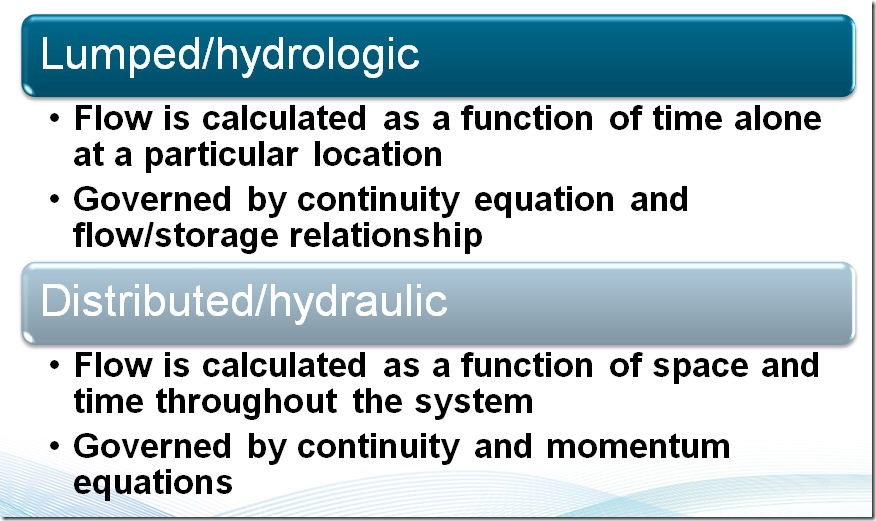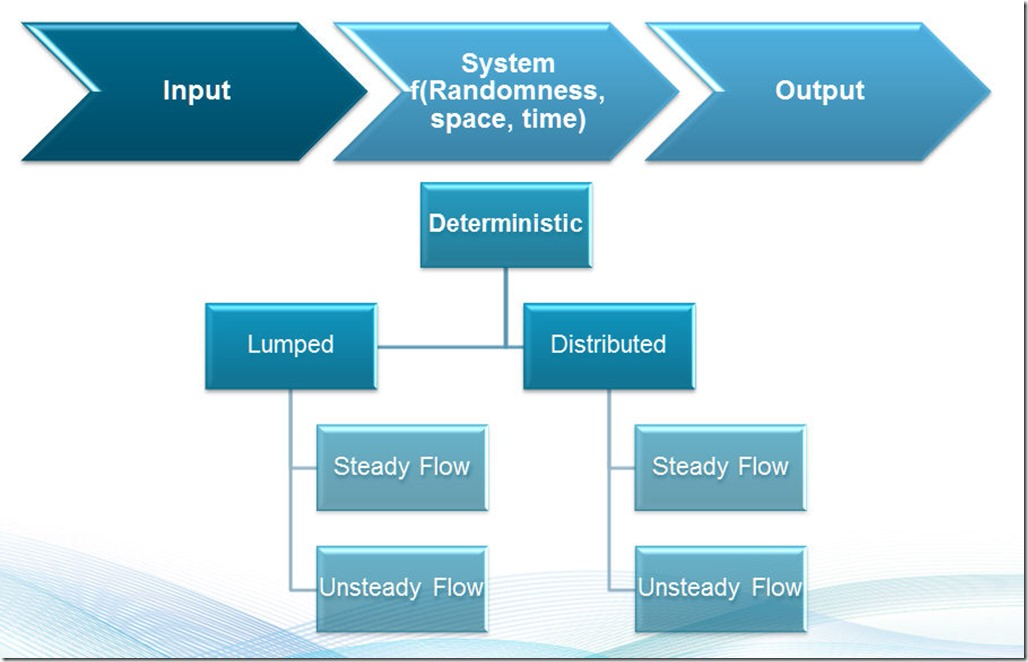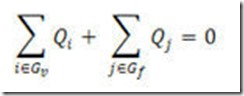### Continuity Equation### Various Forms of the Momentum Equation## 2.  Muskingum-Cunge for InfoSewerH20Map Sewer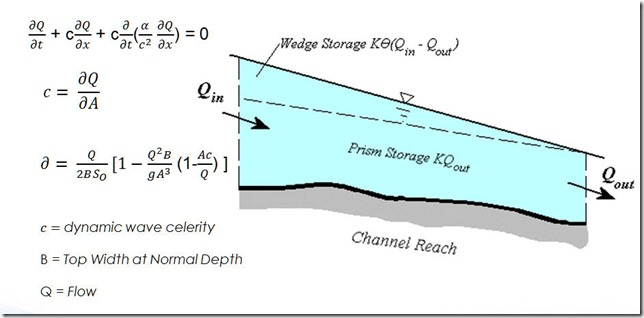The continuity (mass conservation) equation is: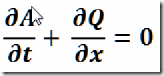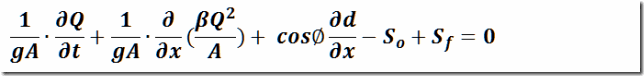where

x          =          distance along the pipe (longitudinal direction of sewer)

A          =          flow cross sectional area normal to x

y          =          coordinate direction normal to x on a vertical plane

d          =          depth of flow of the cross section, measured along y direction

Q         =          discharge through A

V          =          cross sectional average velocity along x direction

S0         =          pipe slope, equal to sin θ

θ          =          angle between sewer bottom and horizontal plane

Sf            =          friction slope

g             =          gravitational acceleration

t           =          time

β          =          Boussinesq momentum flux correction coefficient for velocity distribution

## 3. SWMM5, H2OMap SWMM and InfoSWMM## 4. ICM and ICM SE## 5. A common look at the Equations for ICM, ICM  SE. InfoSWMM and H2OMap SWMM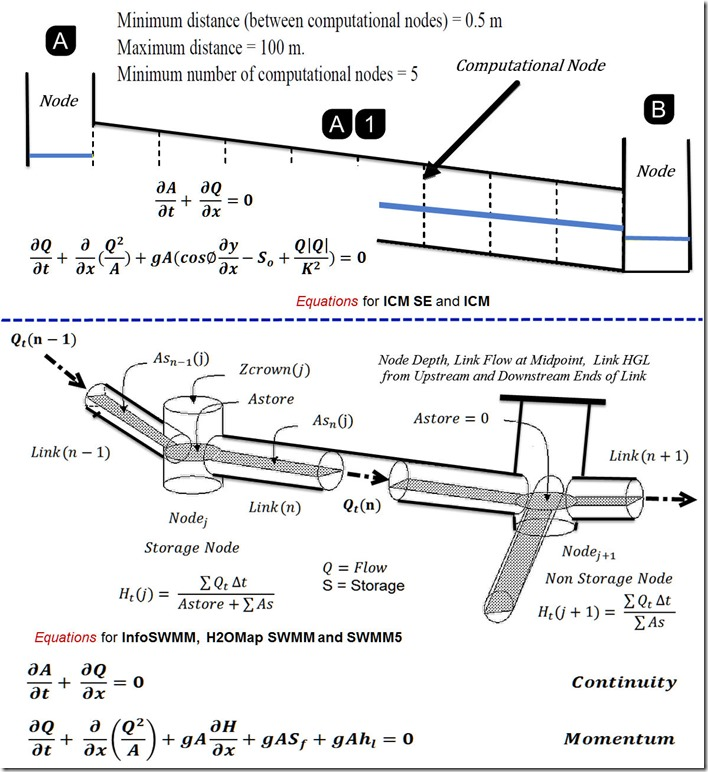## ICM 2D and InfoSWMM 2D Equations

ICM 2D and InfoSWMM share the same computational engine as described on the Innovyze Blog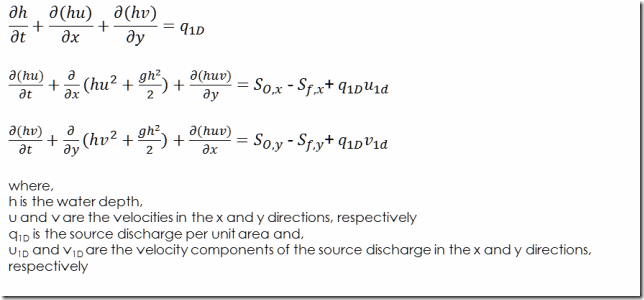As the scheme is an explicit solution it does not require iteration to achieve stability within defined tolerances like the ICM 1D scheme or the iterative solution in InfoSWMM.  Instead, for each element, the required timestep is calculated using the Courant-Friedrichs-Lewy condition in order to achieve stability, where the Courant-Friedrichs-Lewy condition isTranslate »